Courses

# Ex 6.5 NCERT Solutions- Triangles Class 10 Notes | EduRev

## Class 10 : Ex 6.5 NCERT Solutions- Triangles Class 10 Notes | EduRev

The document Ex 6.5 NCERT Solutions- Triangles Class 10 Notes | EduRev is a part of the Class 10 Course Class 10 Mathematics by VP Classes.
All you need of Class 10 at this link: Class 10

Q.1. Sides of triangles are given below. Determine which of them are right triangles. In case of a right triangle, write the length of its hypotenuse.
(i) 7 cm, 24 cm, 25 cm
(ii) 3 cm, 8 cm, 6 cm
(iii) 50 cm, 80 cm, 100 cm
(iv) 13 cm, 12 cm, 5 cm
Sol. (i) 7 cm, 24 cm, 25 cm
(7)2 + (24)2 = 49 + 576
= 625 = (25)2 = 25
∴ The given sides make a right angled triangle with hypotenuse 25 cm
(ii) 3 cm, 8 cm, 6 cm
(8)2 = 64
(3)2 + (6)2 = 9 + 36 = 45
64 ≠ 45
The square of larger side is not equal to the sum of square of other two sides.
∴ The given triangle is not a right angled.
(iii) 50 cm, 80 cm, 100 cm
(100)2 = 10000
(80)2 + (50)2 = 6400 + 2500 = 8900
The square of larger side is not equal to the sum of squares of other two sides.
∴ The given triangle is not a right angled.
(iv) 13 cm, 12 cm, 5 cm
(13)2 = 169
(12)2 + (5)2 = 144 + 25 - 169
Sides make a right angled triangle with hypotenuse 13 cm.

Q.2. PQR is a triangle, right angled at P and M is a point on QR such that PM ⊥ QR. Show that PM2 = QM· MR.
Sol. In right angled ΔQPR,
∠P = 90°, PM QR
∴ ΔPMQ ~ ΔRMP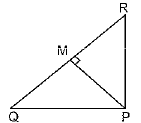[If is drawn from the vertex of right angle to the hypotenuse then triangles on both sides of perpendicular are similar to each other, and to whole triangle]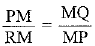[Corresponding sides of similar triangles]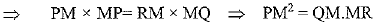Q3. In the figure, ABD is a triangle, right angled at A and AC ⊥ BD. Show that
(i) AB2 = BC.BD
(ii) AC2 = BC.DC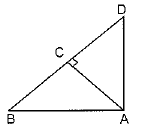Sol. (i) In ΔABC, ∠BAD = 90°
AC BD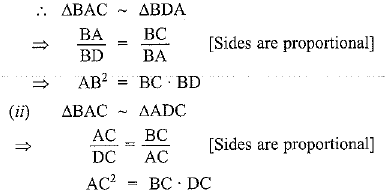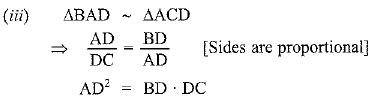Q.4. ABC is an isosceles triangle, right angled at C. Prove that AB2 = 2AC2.
Sol. Given: In ΔABC, ∠C = 90° and AC = BC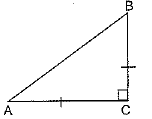To Prove: AB2 = 2AC2
Proof: In ΔABC, AB2 = BC2 + AC2
AB2 = AC2 + AC2 [Pythagoras theorem]
= 2AC2

Q.5. ABC is an isosceles triangle with AC = BC. If AB2 = 2 AC2, prove that ABC is a right triangle.
Sol. Given: In ΔABC, AC = BC and AB2 = 2 AC2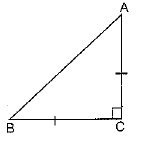To Prove: ACB is a right angled triangle
Proof: AB2 = 2AC2
AB2 = AC2 + AC2
AB2 = AC2 + BC2         [∵ AC = BC]
⇒ ∠ACB = 90°
[By converse of Pythagoras theorem]
∴ ΔABC is right angled triangle

Q.6. ABC is an equilateral triangle of side 2a. Find each of its altitudes.
Sol.  Given: In Δ ABC, AB = BC = AC = 2a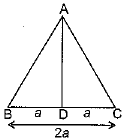Proof: In Δ ABC,
AB = BC = AC = 2a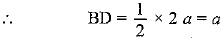⇒ AD2 = AB2 - BD2
= (2a)2 - (a)2 = 4a2 - a2 = 3a2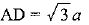Q.7. Prove that the sum of the squares of the sides of a rhombus is equal to the sum of the squares of its diagonals.
Sol. Given: ABCD is a rhombus. Diagonals AC and BD intersect at O.
To Prove: AB2 + BC+ CD+ DA2 = AC2 + BD2
Proof: In ΔAOB, AB2 = AO2 + OB2 [Pythagoras theorem]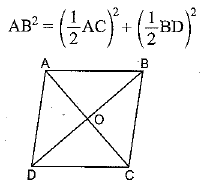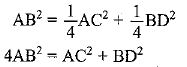AB2 + AB2 + AB2 + AB2 = AC2 + BD2
AB2 + BC2 + CD2 + DA2 = AC2 + BD2
[∵ AB = BC = CD = DA]

Q.8. In the figure, O is a point in the interior of a triangle ABC, OD ⊥ BC, OE ⊥ AC and OF ⊥ AB. Show that
(i) OA2 + OB2 + OC2 - OD2 - OE2 - OF2 = AF+ BD2 + CE2,
(ii) AF2 + BD2 + CE2 = AE2 + CD2 + BF2.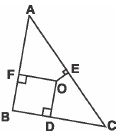Sol. Given: A triangle ABC in which OD ⊥ BC, OE ⊥ AC and OF ⊥ AB
To Prove: (i) OA2 + OB2 + OC2 - OD2 - OE2 - OF2 = AF2 + BD2 + CE2
Construction: Join OA,.OB and OC
Proof: (i) In ΔAOF,
OA2 = OF2 + AF2 [Pythagoras theorem]
AF2 = OA2 - OF2
In ΔBDO, OB2 = BD2 + OD2
BD2 = OB2 - OD2
In ΔCEO, OC2 = CE2 + OE2
CE2 = OC2 - OE2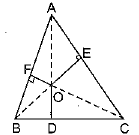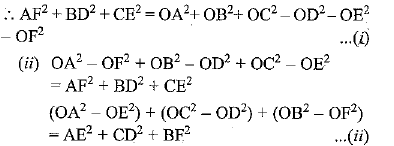Equating (i) and (ii)
AF2 + BD2 + CE2 = AE2 + CD2 + BF2

Q.9. A ladder 10 m long reaches a window 8 m above the ground. Find the distance of the foot of the ladder from base of the wall.
Sol. Let AC be the ladder of length 10 m and AB = 8 m
In ΔABC,
BC2 + AB2 = AC2
⇒ BC2 = AC2 - AB2
= (10)2 - (8)2
BC2 = 100 - 64 = 36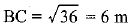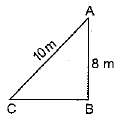Hence distance of foot of the ladder from base of the wall is 6 m.

Q.10. A guy wire attached to a vertical pole of height 18 m is 24 m long and has a stake attached to the other end. How far from the base of the pole should the stake be driven so that the wire will be taut?
Sol. In Δ ABC, AC2 = AB2 + BC2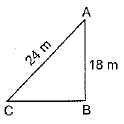⇒ (24)2 = (18)2 + BC2
⇒ 576 = 324 + BC2
⇒ 576 - 324 = BC2
BC2 = 252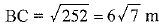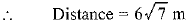Q.11. An aeroplane leaves an airport and flies due north a speed of 1000 km per hour. At the same time, another aeroplane leaves the same airport and flies due west at a speed of 1200 km per hour. How far apart will be the two planes after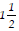hours ?
Sol.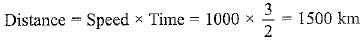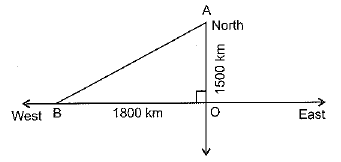Distance travelled by the aeroplane due west in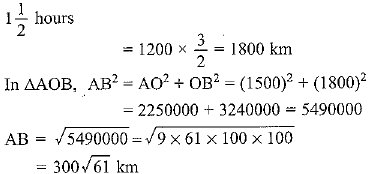Q.12. Two poles of heights 6 m and 11 m stand on a plane ground. If the distance between the feet of the poles is 12 m, find the distance between their tops.
Sol. Length of poles is 6 m and 11m.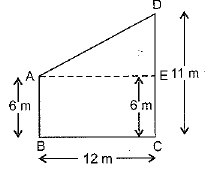DE = DC - EC = 11 m - 6 m = 5 m
In ΔDAE, AD2 = AE2 + DE2    [∴ AE - BC]
= (12)2 + (5)2 = 144 + 25 = 169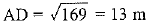Q.13. D and E are points on the sides CA and CB respectively of a triangle ABC, right angled at C. Prove that AE2 + BD2 = AB2 + DE2.
Sol.  In ΔABC, ∠C = 90°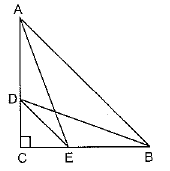AB2 = AC2 + BC2 [Pythagoras theorem]
In ΔDCE, DE2 = DC2+CE2
⇒ AB2 + DE2 = AC2 + BC2 + DC2 + CE2
= AC2 + CE2 + DC2 + BC2
= AE2 + BD2

Q.14. The perpendicular from A on side BC of a Δ ABC intersects BC at D such that DB = 3 CD (see figure). Prove that 2 AB2 = 2 AC2 + BC2.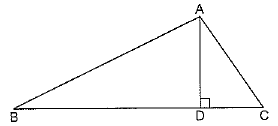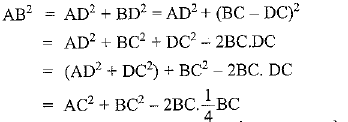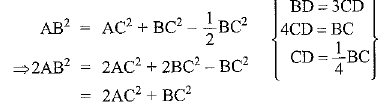Q.15. In an equilateral triangle ABC, D is a point on side BC such that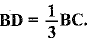Prove that 9 AD2 = 7 AB2.
Sol. Given: In Δ ABC, AB = BC = AC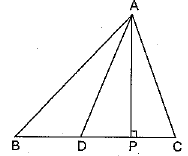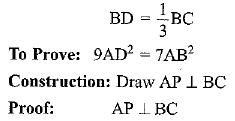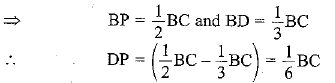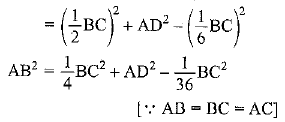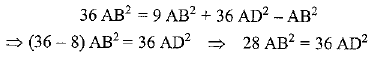On dividing both sides by 4, we get,

Q.16. In an equilateral triangle, prove that three times the square of one side is equal to four times the square of one of its altitudes.
Sol. Given: In ΔABC,
AB = BC = AC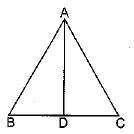To Prove: 3AB2 = 4 AD2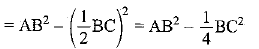⇒ 4AD2 = 4AB2 - BC2
= 4AB2 - AB2 [∴BC = AB = AC]

Q.17. Tick the correct answer and justify: In Δ ABC, AB =  6√3 cm, AC = 12 cm and BC = 6 cm. The angle B is:
(A) 120°
(B) 60°
(C) 90°
(D) 45°
Sol. In ΔABC,
AB = 6√3 cm, AC = 12 cm and BC = 6 cmΔABC is right angled at B.
∴ ∠B = 90°

Offer running on EduRev: Apply code STAYHOME200 to get INR 200 off on our premium plan EduRev Infinity!

132 docs

,

,

,

,

,

,

,

,

,

,

,

,

,

,

,

,

,

,

,

,

,

;